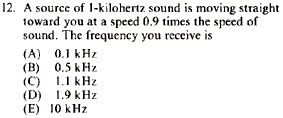GR 8677927796770177 | # Login | Register

GR8677 #12
Problem
 GREPhysics.NET Official Solution Alternate SolutionsWave Phenomena}Doppler Effect

For a source that's coming towards you, its frequency will obviously be higher than its original source frequency. Choices A and B are out. To know for sure whether the choice is C, D, or E, one can apply the Doppler Effect.

The Doppler Effect equation can easily be derived even if one forgets it. A source approaching at would have , the distance between waves, decreasing. . The wave travels at the speed of sound, thus the frequency you receive is: . Choice E.Alternate Solutions
 flyboy6212010-11-09 21:01:01 I find it easier to look at the time between successive wave crests. Let's say if the source were at rest, the time between crests would be . Then if the source is moving toward the observer at , the time between crests is . Therefore the frequency is 10 times higher, as in (E).Reply to this commentkicksp2007-10-29 10:11:11 Quick hand-waving arguments save us time here. Note that the observed frequency goes to infinity as . A simple functional form for (which we could construct immediately even if we forgot the formula) is , yielding (E). Go maroons!Reply to this commentflyboy621
2010-11-09 21:01:01
I find it easier to look at the time between successive wave crests. Let's say if the source were at rest, the time between crests would be . Then if the source is moving toward the observer at , the time between crests is . Therefore the frequency is 10 times higher, as in (E).student2008
2008-10-11 12:48:31
...the frequency of the wave is ....student2008
2008-10-11 12:44:23
The observable phase of the wave front at some point is , where and are the circular frequency and the wave vector measured in the observational reference frame. The source is moving with the constant velocity , so . Thus, . It means that in the reference frame where the source is at rest the frequency of the wave is . Since , we get eventually: .ssp
2008-09-05 02:46:12
Bit more handwaving...

So we take the fact that we know the frequency has to increase from above. Now we look at the speed. The shift has to large because the speed is large (speed and frequency are still proportional) and you got your answer, (E).

Little trick from GRkicksp
2007-10-29 10:11:11
Quick hand-waving arguments save us time here. Note that the observed frequency goes to infinity as . A simple functional form for (which we could construct immediately even if we forgot the formula) is

,

yielding (E).

Go maroons!
 vikhuan2009-06-08 02:04:49 tyjyytyYou are replying to:
Bit more handwaving... So we take the fact that we know the frequency has to increase from above. Now we look at the speed. The shift has to large because the speed is large (speed and frequency are still proportional) and you got your answer, (E). Little trick from GRLaTeX syntax supported through dollar sign wrappers $, ex.,$\alpha^2_0$produces . type this... to get...$\int_0^\infty\partial\Rightarrow\ddot{x},\dot{x}\sqrt{z}\langle my \rangle\left( abacadabra \right)_{me}\vec{E}\frac{a}{b}\$